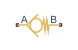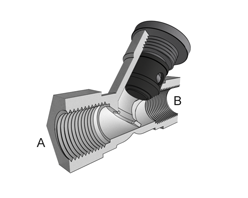# Check Valve (TL)

Valve for the prevention of flow aimed counter to its intended direction

• Library:
• Simscape / Fluids / Thermal Liquid / Valves & Orifices / Directional Control Valves

•## Description

The Check Valve (TL) block models the opening characteristics of a proportional valve that is forced shut when the pressure difference between its ports is reversed (or, more precisely, dropped below a threshold known as the cracking pressure). Check valves are common in backflow prevention devices, such as those used in public water supply networks, where contaminated water downstream of a water main must not be allowed to return upstream. No specific valve shutoff mechanism—whether ball, disc, diaphragm, or other—is assumed in the block. The allowed direction of flow is always from port A to port B.

Y-Shaped Check Valve with Piston-Type Control Member Partially RetractedThe valve cracks open when the pressure drop across it rises above the cracking pressure specified in the block. The opening area increases linearly with pressure, save for two small pressure intervals near the fully open and fully closed positions (over which nonlinear smoothing is applied in order to remove numerical discontinuities). When the pressure drop reaches the maximum value specified in the block, the valve is fully open and its opening area no longer increases with pressure. The flow rate through the valve is never truly zero as a small leakage area remains when the pressure falls below the cracking pressure.

### Valve Opening Area

The (smoothed) valve opening area is first computed as a linear function of pressure, either that at the valve entrance (port A) or the drop between those at the entrance and at the outlet (port B). Which of these pressures—termed control pressures—features in the area calculations depends on the setting of the Pressure specification method block parameter:

where p is pressure; the subscript `Control` indicates the value to be used in determining the opening area of the valve. Subscripts `A` and `B` denote the thermal liquid ports at which the pressures are obtained. The pressures at the ports are always defined as absolute pressures. The cracking pressure, at which the opening area is at a minimum, is similarly defined:

where the subscript `Crack` indicates a valve just cracking open, subscript `G` a gauge value, and subscript `Atm` the standard atmospheric value. The value of pCrack,G is obtained from the Cracking pressure (gauge) block parameter; that of ΔpCrack is obtained from the Cracking pressure differential block parameter. The maximum pressure, at which the opening area is at its largest, is:

where subscript `Max` denotes a maximum pressure (obtained as gauge). The value of pMax,G is obtained from the Maximum opening pressure (gauge) block parameter; that of ΔpMax is obtained from the Maximum opening pressure differential block parameter. The control, cracking, and maximum pressures give for the linear form of the valve opening area:

`${S}_{\text{Lin}}=\frac{{S}_{\text{Max}}-{S}_{\text{Crack}}}{{p}_{\text{Max}}-{p}_{\text{Crack}}}\left({p}_{\text{Ctl}}-{p}_{\text{Crack}}\right)+{S}_{\text{Crack}},$`

where S denotes the (linear) opening area. The opening area at cracking is equal to the small value specified in the Leakage area block parameter. The primary purpose of this parameter is to ensure the numerical robustness of the model by ensuring that no portion of a thermal liquid network becomes completely isolated during simulation.

Valve opening area as a linear function of pressureWhen the valve is in a near-open or near-closed position in the linear parameterization, you can maintain numerical robustness in your simulation by adjusting the parameter. If the parameter is nonzero, the block smoothly saturates the control pressure between pCrack and pMax. For more information, see Numerical Smoothing.

Leakage Area

The primary purpose of the leakage area of a closed valve is to ensure that at no time does a portion of the hydraulic network become isolated from the remainder of the model. Such isolated portions reduce the numerical robustness of the model and can slow down simulation or cause it to fail. Leakage is generally present in minuscule amounts in real valves but in a model its exact value is less important than it being a small number greater than zero. The leakage area is obtained from the block parameter of the same name.

### Mass Balance

The volume of fluid inside the valve, and therefore the mass of the same, is assumed to be very small and it is, for modeling purposes, ignored. As a result, no amount of fluid can accumulate there. By the principle of conservation of mass, the mass flow rate into the valve through one port must therefore equal that out of the valve through the other port:

`${\stackrel{˙}{m}}_{A}+{\stackrel{˙}{m}}_{B}=0,$`

where $\stackrel{˙}{m}$ is defined as the mass flow rate into the valve through one of the ports (A or B).

### Momentum Balance

The causes of those pressure losses incurred in the passages of the valve are ignored in the block. Whatever their natures—sudden area changes, flow passage contortions—only their cumulative effect is considered during simulation. This effect is captured in the block by the discharge coefficient, a measure of the mass flow rate through the valve relative to the theoretical value that it would have in an ideal valve. Expressing the momentum balance in the valve in terms of the pressure drop induced in the flow:

`${p}_{\text{A}}-{p}_{\text{B}}=\frac{{\stackrel{˙}{m}}_{\text{Avg}}\sqrt{{\stackrel{˙}{m}}_{\text{Avg}}^{2}+{\stackrel{˙}{m}}_{\text{Crit}}^{2}}}{2{\rho }_{\text{Avg}}{C}_{\text{D}}{S}_{\text{Smooth}}^{2}}\left[1-{\left(\frac{{S}_{\text{Smooth}}}{{S}_{\text{Lin}}}\right)}^{2}\right]{\xi }_{\text{p}},$`

where CD is the discharge coefficient, and ξp is the pressure drop ratio—a measure of the extent to which the pressure recovery at the outlet contributes to the total pressure drop of the valve. The subscript `Avg` denotes an average of the values at the thermal liquid ports. The critical mass flow rate ${\stackrel{˙}{m}}_{\text{Crit}}$ is calculated from the critical Reynolds number—that at which the flow in the orifice is assumed to transition from laminar to turbulent:

`${\stackrel{˙}{m}}_{\text{Crit}}={\text{Re}}_{\text{Crit}}{\mu }_{\text{Avg}}\sqrt{\frac{\pi }{4}{S}_{\text{Lin}}},$`

where μ denotes dynamic viscosity. The pressure drop ratio is calculated as:

`${\xi }_{\text{p}}=\frac{\sqrt{1-{\left(\frac{{S}_{\text{Smooth}}}{{S}_{\text{Lin}}}\right)}^{2}\left(1-{C}_{\text{D}}^{2}\right)}-{C}_{\text{D}}\frac{{S}_{\text{Smooth}}}{{S}_{\text{Lin}}}}{\sqrt{1-{\left(\frac{{S}_{\text{Smooth}}}{{S}_{\text{Lin}}}\right)}^{2}\left(1-{C}_{\text{D}}^{2}\right)}+{C}_{\text{D}}\frac{{S}_{\text{Smooth}}}{{S}_{\text{Lin}}}}.$`

### Energy Balance

The valve is modeled as an adiabatic component. No heat exchange can occur between the fluid and the wall of the valve. No work is done on or by the fluid as it traverses the valve. With these assumptions, energy can enter and exit the valve by advection only, through ports A and B. By the principle of conservation of energy then, the sum of the energy flows through the ports must always equal zero:

`${\varphi }_{\text{A}}+{\varphi }_{\text{B}}=0,$`

where ϕ is defined as the energy flow rate into the valve through one of the ports (A or B).

## Ports

### Conserving

expand all

Opening through which the flow can enter the valve.

Opening through which the flow can exit the valve.

## Parameters

expand all

Choice of pressure to use in the block calculations. The default setting corresponds to the gauge pressure at the valve inlet (port A). The alternative setting corresponds to the pressure differential from inlet (A) to outlet (B).

Gauge pressure at port A at which the valve begins to open. The valve opening area is saturated at the value of the Leakage area block parameter when the pressure at port A is at or below the value specified here.

#### Dependencies

This parameter is active when the Pressure control specification block parameter is set to `Pressure at port A`.

Gauge pressure at port A at which the valve is fully open. The valve opening area is saturated at the value specified in the Maximum opening area block parameter when the pressure at port A is at or above the value specified here.

#### Dependencies

This parameter is active when the Pressure control specification block parameter is set to `Pressure at port A`.

Pressure differential from port A to port B at which the valve begins to open. The valve opening area is saturated at the value of the Leakage area block parameter when the pressure differential is at or below the value specified here.

#### Dependencies

This parameter is active when the Pressure control specification block parameter is set to `Pressure differential`.

Pressure differential from port A to port B at which the valve is fully open. The valve opening area is saturated at the value specified in the Maximum opening area block parameter when the pressure differential is at or above the value specified here.

#### Dependencies

This parameter is active when the Pressure control specification block parameter is set to `Pressure differential`.

Opening area of the valve in the fully open position. The valve is fully open if the pressure drop from port A to port B is equal to or greater than that given by the Maximum opening pressure block parameter.

Opening area of the valve in the fully closed position, when only internal leakage between its ports remains. This parameter serves primarily to ensure that closure of the valve does not cause portions of the thermal liquid network to become isolated. The exact value specified here is less important than its being a small number greater than zero.

Measure of the amount of smoothing to apply to the opening area function. This parameter determines the widths of the regions to be smoothed, one being at the fully open position, the other at the fully closed position. The smoothing superposes on the linear opening area function two nonlinear segments, one for each region of smoothing. The greater the value specified, the greater the smoothing and the broader the nonlinear segments.

Area normal to the flow path at each port. The ports are assumed to be equal in size. The flow area specified here should match those of the inlets of those components to which the orifice connects.

Ratio of the actual flow rate through the valve to the theoretical value that it would have in an ideal valve. This semi-empirical parameter measures the flow allowed through the valve: the greater its value, the greater the flow rate. Refer to the valve data sheet, if available, for this parameter.

Reynolds number at which the flow is assumed to transition between laminar and turbulent regimes.

Variables

Desired mass flow rate into the valve through port A at the start of simulation. This parameter serves as an initial state target, a guide used by Simscape in assembling the initial configuration of the model. How closely the target is met depends on the constraints imposed by the remainder of the model and on the priority level specified.

## Version History

Introduced in R2016a